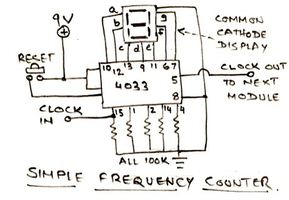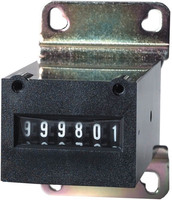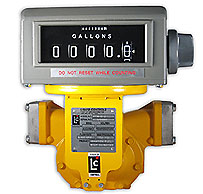I’ve been given a nice puzzle that I think is a good blog article opportunity, as it involves some thinking and window functions.

# Ever Increasing Counter, with Resets

Say we store in a table entries from a counter that only increases and the time stamp when we did the measurement. So that when you read `30` then later `40` in fact that means we counted `10` more the second reading when compared to the first, in other words the first `30` are counted again in the second counter value, `40`.

Now of course it’s a real world counter. Think network traffic counter on a network interface, if you want something real to play with in your mind. So the counter will sometime reset and you will read measure sequences such as `40, 0, 20` if you happen to read just when the counter is reset, or most of the time that will look like `45, 25, 50`.

The question we want to answer is, given a series of that counter measures, including some resets, what is the current logical value of the counter?

Given the sequence of measures `0, 10, 20, 30, 40, 0, 20, 30, 60` the result we want is `40 + 60`, that is `100`. Right?

# Playing with some data

Let’s model an hypothetical dataset easy enough to play with. What about just the previous example? We also need to time stamp the measurements, let’s just use a tick for now, as it’s easier to think about:

``````create table measures(tick int, nb int);

insert into measures
values (1, 0), (2, 10), (3, 20), (4, 30), (5, 40),
(6, 0), (7, 20), (8, 30), (9, 60);
``````

Now that we have some data in a table to play with, let’s try to find out the numbers we are interested in: we only want to keep the latest measure we read on the counter just before it wraps. That means values where the next one (in tick or time stamping order) is lesser than the current counter value.

As we are lucky enough to be playing with the awesome PostgreSQL which brings window functions on the table, we can easily implement just what we said in a readable way:

``````select tick, nb,
case when lead(nb) over w < nb then nb
when lead(nb) over w is null then nb
else null
end as max
from measures
window w as (order by tick);
``````

The firt case is the exact translation of the problem as spelled in english in just the previous paragraph where we stated we want to keep the current counter value in case of a wraparound, so I guess it’s easy enough to get at.Then we have a couple of tricks in that query in order to massage the data as we want it. First, the last row of the output won’t have a lead, that window function call is going to return `NULL`. In that case, we keep the current counter value as if we just did a wraparound. And finally, when there’s no wraparound, we don’t care about the data. Well, for the purpose of knowing the current logical value of the counter, that is.

And we get that encouraging result:

`````` tick | nb  | max
------+-----+-----
1 |   0 |
2 |  10 |
3 |  20 |
4 |  30 |
5 |  40 |  40
6 |   0 |
7 |  20 |
8 |  30 |
9 |  60 |  60
``````

As you see, we have been able to create a new column out of the dataset, and that new column only contains the data we are interested into.

# Finding the current counter value

All we have to do now is sum this computed columns entries. Remember that the `sum()` aggregate function will simply discard nulls, so that we don’t have to turn them into a bunch of `0`.

``````with t(tops) as (
select case when lead(nb) over w < nb then nb
when lead(nb) over w is null then nb
else null
end as max
from measures
window w as (order by tick)
)
select sum(tops) from t;
``````

And here’s the expected result:

`````` sum
-----
100
``````

Now what about testing with another set of data or two, just to be sure that the counter is allowed to wrap more than once within our solution?

``````insert into measures
values (10, 0), (11, 10), (12, 30), (13, 35), (14, 45),
(15, 25), (16, 50), (17, 100), (18, 110);
``````

Then when running the same with t(tops) query again this time we get the following result:

`````` sum
-----
255
(1 row)
``````

All good!

# Counter logical value over a given periodNow of course what we want is to find the logical value of the counter for a given day’s or month’s worth of measures. We then need to pay attention to the value of the counter at the start of our period so that we know to substract it from the logical sum over the period.

Here’s an SQL version of the same sentence, applied to the period in between ticks `4` and `14`, in a completely arbitrary choosing of mine:

``````with t as (
select tick,
first_value(nb) over w as first,
case when lead(nb) over w < nb then nb
when lead(nb) over w is null then nb
else null
end as max
from measures
where tick >= 4 and tick < 14
window w as (order by tick)
)
select sum(max) - min(first) as sum
from t;
``````

Here we are using the first_value() window function to retain it in the whole resultset of the Common Table Expression (the inner query introduced by the keyword `WITH` is called that way). And when doing the sum we’re interested in at the outer level, we didn’t forget to substract the first value: we need to use an aggregate here because we’re doing a `sum()` aggregate at the same query level, and we have the same value in each row of the resultset, so we used `min()`, `max()` would have been as good.

Another important trick we’re using in that query is how to express the date range. Never use `between` for that, as you would end up counting boundaries twice, and customer won’t like your accounting process if you do that. Always use a combo of inclusive and exclusive boundaries comparison, as in that `WHERE` clause in the previous query.

Let’s have a quick look at the raw data in that range, using another nice aggregate that PostgreSQL comes with:

``````select array_agg(nb) from measures where tick >= 4 and tick < 14;
array_agg
-------------------------------
{30,40,0,20,30,60,0,10,30,35}
(1 row)
``````

And now, the logical counter value for that period is computed as the following value by the previous query:

`````` sum
-----
105
(1 row)
``````

We can verify it manually, we want `40 + 60 + 35 - 30`, I think we’re all good again. Don’t forget we have to substract the first measure from the period!

# Extending the problem

Another interesting problem, that we didn’t have here but that I find interesting enough to extend this article, is finding the ranges of time (here, ticks) within which the counter didn’t reset.The query is more complex because we need to split the data into partitions, each partition containing data from the same counter series of measures without wrapping. The usual trick is to self-join our data set so that for each given row we have a set of rows from the same partition, we are going to instead use a correlated subquery to go fetch the next wraparound value:

``````with tops as (
select tick, nb,
case when lead(nb) over w < nb then nb
when lead(nb) over w is null then nb
else null
end as max
from measures
window w as (order by tick)
)
select tick, nb, max,
(select tick
from tops t2
where t2.tick >= t1.tick and max is not null
order by t2.tick
limit 1) as p
from tops t1;
``````

Which results in the following partitioned output:

`````` tick | nb  | max | p
------+-----+-----+----
1 |   0 |     |  5
2 |  10 |     |  5
3 |  20 |     |  5
4 |  30 |     |  5
5 |  40 |  40 |  5
6 |   0 |     |  9
7 |  20 |     |  9
8 |  30 |     |  9
9 |  60 |  60 |  9
10 |   0 |     | 14
11 |  10 |     | 14
12 |  30 |     | 14
13 |  35 |     | 14
14 |  45 |  45 | 14
15 |  25 |     | 18
16 |  50 |     | 18
17 | 100 |     | 18
18 | 110 | 110 | 18
(18 rows)
``````

With that as an input it’s then possible to build ranges of ticks including non wrapping set of measures from our counter, and get for each range the logical value tat the counter had at the end of it:

``````with tops as (
select tick, nb,
case when lead(nb) over w < nb then nb
when lead(nb) over w is null then nb
else null
end as max
from measures
window w as (order by tick)
),
parts as (
select tick, nb, max,
(select tick
from tops t2
where t2.tick >= t1.tick and max is not null
order by t2.tick
limit 1) as p
from tops t1
),
ranges as (
select first_value(tick) over w as start,
last_value(tick) over w as end,
max(max) over w
from parts
window w as (partition by p order by tick)
)
select * from ranges where max is not null;
``````

And there we have a single row per partition, with the start and end values for the ticks, and the counter’s proper max value:

`````` start | end | max
-------+-----+-----
1 |   5 |  40
6 |   9 |  60
10 |  14 |  45
15 |  18 | 110
(4 rows)
``````

# Conclusion

What I hope to have shown here, apart from some window function tips and some nice use cases for common table expressions, is that as a developper adding `SQL` to your tool set is a very good idea.You don’t want to have several parts of your code dealing with a logical counter like this, because you want the reporting, accounting, quota, billing and other software to all agree on the values. And you most probably want to avoid to fetch a huge result set of data and process it in the application memory (it’d better fit) rather than just get back a single integer column single row resultset, right?

If you find this SQL example to be off the limits, it’s a good sign that you need to improve on your skills so that SQL is a real asset of your developer multi languages multi paradygm talents.